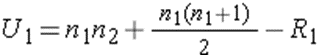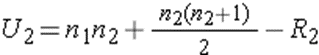We use cookies to provide you with a better experience. You can read our Cookie Policy here.Article

# Mann-Whitney U Test: Assumptions and ExampleArticle

# Mann-Whitney U Test: Assumptions and Example

Complete the form below and we will email you a PDF version of "Mann-Whitney U Test: Assumptions and Example "

First Name*
Last Name*
Country*
Company Type*
Job Function*
Would you like to receive further email communication from Technology Networks?

Technology Networks Ltd. needs the contact information you provide to us to contact you about our products and services. You may unsubscribe from these communications at any time. For information on how to unsubscribe, as well as our privacy practices and commitment to protecting your privacy, check out our Privacy Policy

## What is the Mann-Whitney U Test?

The Mann-Whitney U Test, also known as the Wilcoxon Rank Sum Test, is a non-parametric statistical test used to compare two samples or groups.

The Mann-Whitney U Test assesses whether two sampled groups are likely to derive from the same population, and essentially asks; do these two populations have the same shape with regards to their data? In other words, we want evidence as to whether the groups are drawn from populations with different levels of a variable of interest. It follows that the hypotheses in a Mann-Whitney U Test are:

• The null hypothesis (H0) is that the two populations are equal.
• The alternative hypothesis (H1) is that the two populations are not equal.

Some researchers interpret this as comparing the medians between the two populations (in contrast, parametric tests compare the means between two independent groups). In certain situations, where the data are similarly shaped (see assumptions), this is valid – but it should be noted that the medians are not actually involved in calculation of the Mann-Whitney U test statistic. Two groups could have the same median and be significantly different according to the Mann-Whitney U test.

## When to use the Mann-Whitney U Test

Non-parametric tests (sometimes referred to as ‘distribution-free tests’) are used when you assume the data in your populations of interest do not have a Normal distribution. You can think of the Mann Whitney U-test as analogous to the unpaired Student’s t-test, which you would use when assuming your two populations are normally distributed, as defined by their means and standard deviation (the parameters of the distributions).Figure 1: Normal distribution versus skewed distribution

The Mann-Whitney U Test is a common statistical test that is used in many fields including economics, biological sciences and epidemiology. It is particularly useful when you are assessing the difference between two independent groups with low numbers of individuals in each group (usually less than 30), which are not normally distributed, and where the data are continuous. If you are interested in comparing more than two groups which have skewed data, a Kruskal-Wallis One-Way analysis of variance (ANOVA) should be used.

Mann-Whitney U Test Assumptions

Some key assumptions for Mann-Whitney U Test are detailed below:

• The variable being compared between the two groups must be continuous (able to take any number in a range – for example age, weight, height or heart rate). This is because the test is based on ranking the observations in each group.
• The data are assumed to take a non-Normal, or skewed, distribution. If your data are normally distributed, the unpaired Student’s t-test should be used to compare the two groups instead.
• While the data in both groups are not assumed to be Normal, the data are assumed to be similar in shape across the two groups.
• The data should be two randomly selected independent samples, meaning the groups have no relationship to each other. If samples are paired (for example, two measurements from the same group of participants), then a paired samples t-test should be used instead.
• Sufficient sample size is needed for a valid test, usually more than 5 observations in each group.

## Mann-Whitney U Test Example

Consider a randomized controlled trial evaluating a new anti-retroviral therapy for HIV. A pilot trial randomly assigned participants to either the treated or untreated groups (N=14). We want to assess the viral load (quantity of virus per milliliter of blood) in the treated versus the untreated groups. In practice, a Mann-Whitney U Test would be easily and quickly calculated using statistical software such as SPSS or Stata, but the steps are laid out below.

The data are shown below:

 Treated 540 670 1000 960 1200 4650 4200 Untreated 5000 4200 1300 900 7400 4500 7500

These data are both skewed with a sample size of n=7 in each treatment arm, and so a non-parametric test is appropriate. Before we calculate the test, we choose a significance level (usually α=0.05). The first step is to assign ranks to the values from the full sample (both treatment groups pooled together) in order from smallest to largest. We can then generate a test statistic based on the ranks.

The table below shows the viral load values in the treated and untreated groups ranked smallest to largest, along with the summed ranks of each group:

 Viral load (Treated) Viral load (Untreated) Rank (Treated) Rank (Untreated) 540 1 670 2 900 3 960 4 1000 5 1200 6 1300 7 4200 8 4500 9 4650 10 5000 11 6100 12 7400 13 7500 14 R1=36 R2=69

After summing the ranks for each group, the Mann-Whitney U test statistic is selected as the smallest of the two following calculated U values:Where we let 1 denote the treated group and 2 denote the untreated group (denotation of groups is arbitrary), where n1 and n2 are the number of participants and where R1 and R2 are the sums of the ranks in the treated and untreated groups, respectively. In this example, U1=41 and U2=8. We therefore select U=8 as the test statistic.

## Normal approximation

There are situations where the sample size may be too large for the reference table to be used to calculate the exact probability distribution – in which case we can use a Normal approximation instead. Since U is found by adding together independent, similarly distributed random samples, the central limit theorem applies when the sample is large (usually >20 in each group). The standard deviation of the sum of the ranks can be used to generate a z-statistic and a significance value generated this way. If the null hypothesis is true, the distribution of U approximates to a Normal distribution.

Next we determine a ‘critical value’ of U with which to compare our calculated test statistic, which we can do using a reference table of critical values and using our sample sizes (n=7 in both groups) and two-sided level of significance (α=0.05).

In our current example, the critical value can be determined from the reference table as 8. Finally, we can use this to accept or reject the null hypothesis using the following decision rule: Reject H0 if U ≤ 8.

Given that our U statistic is equal to the critical value, we can reject the null hypothesis that the two groups are equal and accept the alternative hypothesis that there is evidence of a difference in viral load between the groups treated with the new therapy versus untreated.

Elliot McClenaghan is a research fellow in Epidemiology and Medical Statistics at the London School of Hygiene & Tropical Medicine